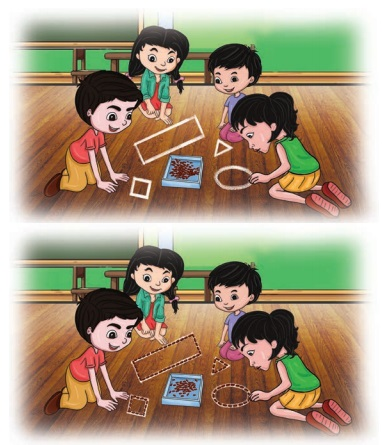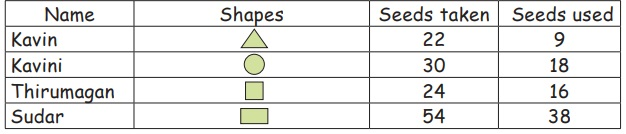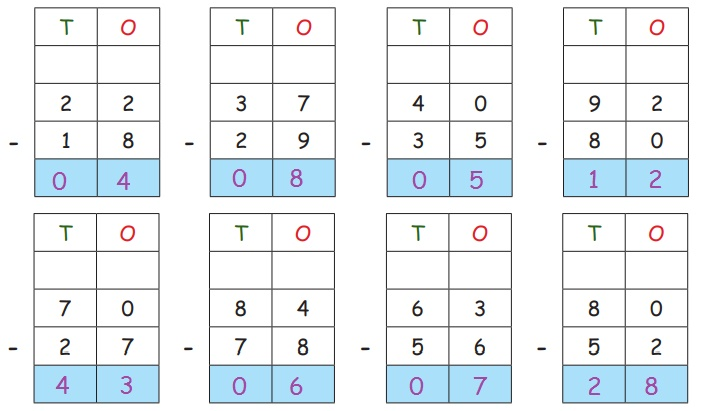Home | | Maths 2nd Std | Subtraction upto 99 with regrouping

# Subtraction upto 99 with regrouping

Keywords: Subtract, Difference, Balance

Subtraction upto 99 with regrouping

Recall

i) Subtract the following two-digit numbers.ii) Two squirrels collected nuts in the hollow of tree trunk.

Listen to their conversation.Can you help the squirrels to find the number of nuts that are left?

Total number of nuts = 58

To find the remaining number of nuts at the end of the first day, we need to subtract 12 from 58.Continue subtracting successively for finding the number of nuts that are left at the end of second and third day complete the subtraction procedure.

Travel Through

Each of the four friends Kavin, Kavini,Thirumagan and Sudar collected tamarind seeds and made geometrical shapes using the seeds.

Keywords: Subtract, Difference, BalanceThe number of seeds used by them are tabulated as follows:Kavin takes 22 seeds and used 9 seeds for making a triangle.

How many seeds are left with him? 13 seeds left

Sudar takes 54 seeds and used 38 seeds to make a rectangle. Find the number of seeds left with her? 16 seeds left

Teacher’s Note: Teacher can motivate children to frame different subtraction questions and subtract them using the information given in the above table.

Learn

Subtract using seeds

Kavin make a triangular shape using the seeds collected by him. Observe the table, write the subtraction fact to find the number of seeds left with him.

To find the number of seeds left with Kavin, we need to subtract the number of seeds used from the number of seeds taken by him.

Let us subtract 9 from 22 using the seeds followed by the subtraction procedure. Take 22 seeds into groups of tens and ones.

Step 1: Subtract the ones

Represent 22 as 2 tens and 2 ones and cancel 9 seeds from ones.Step 2: Regroup by converting tens to ones

We need to take away 9 seeds so we subtract the ones. We cannot subract 9 ones from 2 ones. So, we should regroup the seeds to overcome the shortage of seeds. For that 1 ten is changed into 10 ones.Step 3: Subtract the ones

Subtract 9 ones from 12 ones.Step 4 : Subtract the tens.

Now subtract tens. There is no tens to subtract. So 1 ten is to be written.Hence, the number of seeds left with Kavin is 13.

Learn

Subtraction using abacus

Sudar collected 54 seeds and used 38 seeds to form a rectangle.

How many seeds are left with her?

To find the number of seeds left with Sudar we shall subract 38 from 54. We can do the subtraction 54-38 using abacus followed by the subtraction procedure. 54 has 5 tens and 4 ones.

So, put  5 green beads in tens place and 4 red beads in ones place. To take away 38 from 54 we need to take away 3 tens and 8 ones from 5 tens and 4 ones.Step 1: Subtract ones

We cannot subtract 8 ones from 4 ones.

So exchange 1 ten as 10 ones.

So we haveStep 2: Cancel 8 ones from 14 ones

Now we can subtract 8 ones from 14 ones.Step 3: Subtract tens

Subtract 3 tens from 4 tens.So, the number of beads left with Sudar is 16.

Practice

1. How many more seeds were used for making rectangle than triangle ?2. Thirumagan collected 24 seeds and used 16 seeds. How many seeds are left with him?24 – 16 = 8

3. Kavini used 18 out of 30 seeds collected by her. What is the difference?30−18=12

4. What is the difference in the number of seeds used by kavin and kavini?

Kavini: 18 seeds

Kavin: 9

Difference: 18 – 9 = 9Practice

Subtract the following two-digit numbers.32 – 28 =  4

94 – 59 = 35

72 – 46 = 26

46 – 17 = 29

50 – 36 = 14

85 – 67 = 18

Think Like a Mathematician

Write the missing numbers in the boxes below.29 + 0 = 29,

29 − 0 = 29

Try This

Find the missing digits in each of the subtraction facts.Know more

i) We can check the subtraction process in two ways.

Consider the subtraction fact 53 – 36=17.

i) By adding: 36 + 17 = 53

ii) By subtracting: 53 – 17 = 36

Given two numbers 53 and 36. When 36 is subracted from 53, we get 17. To verify the answer we can either add the number 36 and 17 to get the given number 53 or subract 17 from 53 to get the other number 36.

ii) When we subtract 0 from any number we get the same number.It means we did not take away anything from the number.

iii) When a number is subtracted from itself it gives 0.It means we have taken away completely from the given number.

Mental maths

1. Kamal fixed 56 tubelights in a party hall. If 18 tubelights were fused, then how many tubelights were used?

Ans :56 − 18 =  38 tubelight

2. A factory produced 95 cars in a day. How many cars were unsold if 37 were sold?95 − 37 = 58 cars

3. Kumar walked 23 steps out of 90 steps to reach a shop. How many more steps he has to walk to reach the shop?23 − 90 = 67 steps

4. Kamali had 31 rupees with her. She bought a pen for 15 rupees. She saved the remaining amount. Find the amount saved by her.31 − 15 = 16 rupees

5. In a class of 42 students, 26 are girls. How many are boys?42 − 26 = 16 boys

Tags : Numbers | Term 2 Chapter 2 | 2nd Maths , 2nd Maths : Term 2 Unit 2 : Numbers
Study Material, Lecturing Notes, Assignment, Reference, Wiki description explanation, brief detail
2nd Maths : Term 2 Unit 2 : Numbers : Subtraction upto 99 with regrouping | Numbers | Term 2 Chapter 2 | 2nd Maths